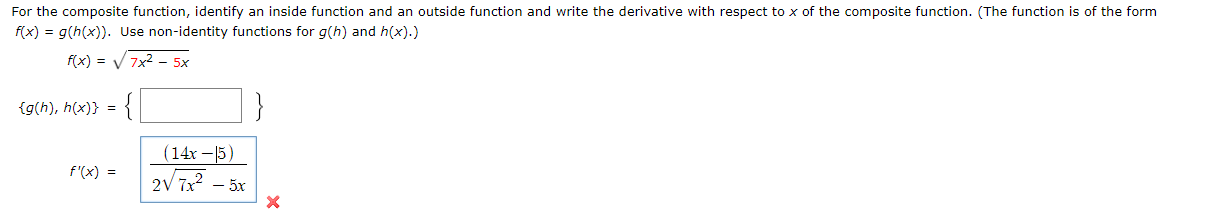# For the composite function, identify an inside function and an outside function and write the derivative with respect to x of the composite function. (The function is of the formfx) g(h(x)) Use non-identity functions for g(h) and h(x).)f(x) 7x2 -5x{{g(h), h(x)}(14x-5)f'(x)2V 7x2-5xX

Questionhelp_outlineImage TranscriptioncloseFor the composite function, identify an inside function and an outside function and write the derivative with respect to x of the composite function. (The function is of the form fx) g(h(x)) Use non-identity functions for g(h) and h(x).) f(x) 7x2 -5x { {g(h), h(x)} (14x-5) f'(x) 2V 7x2-5x X fullscreen
check_circleExpert Solution
Step 1

The given composite function is,

Step 2

The following functions can be considered as the inside and outside function.

Step 3

Thus, the required composition func...

### Want to see the full answer?

See Solution

#### Want to see this answer and more?

Solutions are written by subject experts who are available 24/7. Questions are typically answered within 1 hour*

See Solution
*Response times may vary by subject and question
Tagged in

### Functions